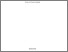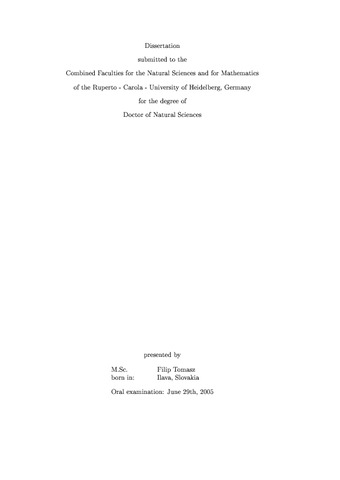## Investigation of Exclusive Photoproduction of eta-rho0 and pi0-rho0 Meson Pairs at HERA

Tomasz, Filip

German Title: Untersuchung der exklusiven Photoproduktion von eta-rho0 und pi0-rho0 Mesonpaaren bei HERAPreview
Postscript, English
Exclusive photoproduction of $\eta-\rho^{0}$ and $\pin-\rho^{0}$ meson pairs is studied with the H1 detector at HERA. The analysis is based on data recorded in 1996-1997 and 1999-2000 data taking periods, corresponding to an integrated luminosity of $76.21\,\,{\tt pb}^{-1}$. %{/pb}$. The$\eta$and$\pin$mesons are identified via their photonic decays in the backward calorimeter SpaCal, and the$\rho^{0}$meson via its dominant decay into$\pip\pim$pairs in the central tracking system of the H1 detector. The scattered proton or its remnant is not measured but is separated by a large rapidity gap from the$\rho^{0}$meson. \\ The cross section for photoproduction of$\eta-\rho^{0}$meson pairs is measured at an average photon-proton center of mass energy of$W\approx208\,{\tt GeV}$in the kinematical region defined by the rapidities of the$\eta$and$\rho^{0}$mesons in the laboratory system:$-3.5<Y^{lab}_{\eta}<-2$and$-1.5<Y^{lab}_{\rho^{0}}<1.5$, photon virtualities$Q^{2}<0.01\,{\tt GeV}^{2}$and inelasticity$0.3<y<0.65$. The cross section obtained is$\sigma^{*}(\gamma p\rightarrow\eta\rho^{0} X) = (3.5 \pm 1.4 (stat) \pm 1.3 (syst))\,\,\, {\tt nb}$. Due to the limited statistics no definitive statement is made to explain this process but the measured differential distributions are consistent with a contribution from Pomeron exchange.\\ In the analysis of photoproduced$\pin-\rho^{0}$meson pairs no significant$\rho$meson signal can be extracted. % the signal of the$\rho^{0}$meson can not exctracted. Therefore only an upper limit of the cross section is given as$\sigma^{*}(\gp\to\pin \, \rho^{0} \, X) \, < \, 8.6 \,\,\,{\tt nb}$at$95\,\%$confidence level, measured at an average photon-proton center of mass energy of$W\approx180\,{\tt GeV}$in the kinematical region defined by the rapidities of the$\pin$meson and the$\pip\pim$pair in the laboratory system:$-3.5<Y^{lab}_{\pin}<-2$and$-1.5<Y^{lab}_{\pim\pip}<1.5$, photon virtualities$Q^{2}<0.01\,{\tt GeV}^{2}$, and inelasticity$0.3<y<0.65\$.